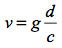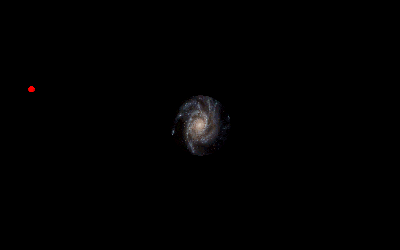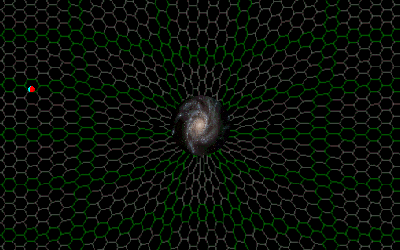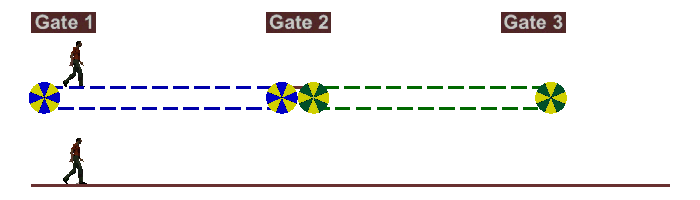"The important thing in science is not so much to obtain new facts as to discover new ways of thinking about them."

- Sir William Bragg -

## Gravitational Lensing

As a spaceship flies by a massive object, its direction will be altered by its gravitational attraction to the object. Similarly, a light ray's path will become deflected as it passes by a massive object (such as a sun, a galaxy or galaxy cluster). This is predicted by Newton's law of gravitation, which says that a photon will follow the same path as a free falling object, thereby causing its path to be slightly altered.The downward acceleration of an object or photon is given by:The velocity of an object is defined by the equation: v = d/t. The equation for the velocity of a photon of light is: c = d/t. Therefore the duration of time that a photon falls would be: t = d/c. The velocity of an accelerating object is: v = at or
v = gt. Therefore the downward velocity the photon acquires is given by:Substitute in equation (1) we get:Referring to the above figure we can calculate the angle of deflection (in radians) by using tangent function where:The total deflection will be twice this amount since the photon will experience the same deflection coming towards the massive object as when leaving.Where:

G is the Gravitational Constant

M is the mass of the attracting object

d is the distance from the center of the object

c  is the speed of light

A simulation of this deflection is demonstrated in Figure 27.Figure 27

However, Einstein's general theory of relativity predicts that a photon will actually be deflected by twice the amount that Newton predicted. Unlike Newtonian space that is assumed to be flat and featureless, general relativity describes space (and time) to be curved or distorted. This 'curvature of space-time' causes an additional deflection, given by the equation:The red photon in Figure 28 shows the path a photon will take as predicted by Newton's laws. The cyan colored photon shows the path a photon will take as predicted by Einstein's general theory of relativity. The latter deflection was verified by Sir Arthur Eddington in 1919 during a solar eclipse.

Not only is there a greater deflection, but the photon is delayed as well. This delay was successfully measured in 1964 by Irwin I. Shapiro by bouncing radar signals off the planets Venus and Mercury.

I have added a foamy ether background to Figure 28 to show how a massive object (such as a galaxy) distorts the space around it. You can see that the size of the hexagonal cells become smaller as you get closer to the galaxy. This is why a passing photon experiences additional deflection and delay; it takes a fixed amount of time for a photon to traverse a foamy ether 'cell'. The smaller cells in the photon's path will cause a delay. This delay causes the photon to 'hang around' the galaxy a little longer, thereby giving the galaxy's gravitational field more time to act on the photon.

Figure 27Figure 28

Foamy ether theory predicts an even greater deflection when dealing with very large objects such as galaxies and galaxy clusters. This additional deflection has already been measured by the astrophysics community in the form of gravitational lensing. Astronomers can calculate the mass of a galaxy (or galaxy cluster) by measuring the amount of deflection (or lensing) that takes place. What astronomers have recently discovered is that the amount of lensing is far greater than what can be accounted for by just measuring the visible mass of the galaxies. This has lead the astrophysics community to conclude that the galaxy must contain some (yet to be discovered) invisible matter, or dark matter.

The inflow that foamy ether theory uses to describe gravity can be used to explain this additional lensing, rather than using dark matter as an explanation. I have created a simulation in Figure 29 to help explain how inflow can cause additional deflection (or lensing). Imagine that the two men in the simulation below are at an airport. The man on the top is walking on a moving sidewalk (like you see in many large airports), and the man on the bottom is walking on a plain floor without any assistance. Both men start at the same time and place (Gate 1) and both walk at exactly the same speed. Between gate 1 and gate 2, the top man is walking in the same direction as the moving sidewalk, so he gets to gate 2 sooner (because his total speed is his speed plus the speed of the sidewalk). But if when the top man passes gate 2, he transfers onto a sidewalk that is moving in the opposite direction to his (his total speed will be his speed minus the speed of the sidewalk). Because his total speed is now less, the bottom man will eventually overtake him and end up reaching gate 3 sooner. This moving sidewalk analogy can be used to model ether inflow.Figure 29

Similar to the men in Figure 29, the photons in Figure 30 start out at the same time and place (the red and cyan photons from Figure 14 & 15 are used for comparison). I have added a foamy ether background and included the inflow that is described in foamy ether theory. If you follow the green photon now, you can see that it initially overtakes the red and cyan photons while it is approaching the galaxy, because it is going with the flow of ether (similar to the top man in Figure 29 traveling from gate 1 to gate 2). But after the green photon passes the center point of the galaxy, it is traveling against the flow of ether (like the top man traveling from gate 2 to gate 3). Since this causes the green photon to 'hang around' the galaxy a little longer, it is influenced by the galaxy's gravitational pull for a longer period of time. This causes an additional amount of deflection and delay over and above that which is accounted for by Newtonian law and general relativity theory!

This extra deflection would be negligible around smaller objects such as our sun because it only takes 4.64 seconds for a photon to traverse the width of the sun. The few seconds that a photon is in close proximity to the sun is hardly enough for the ether inflow to make a noticeable difference. Since galaxies and galaxy clusters are thousands or millions of light years across, a photon traversing it would have plenty of time to be affected by the ether inflow.

So foamy ether theory states that it is the ether inflow that causes the additional deflection (or gravitational lensing). It is my belief that cosmologists have erroneously concluded that the extra deflection is caused by some extra (unknown, dark) matter.

Foamy Ether Theory states that dark matter is unnecessary.Figure 30